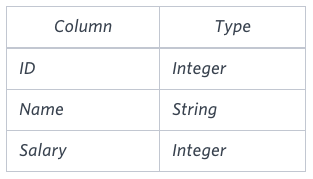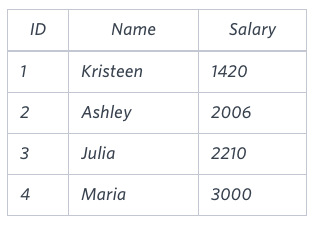## Algorithm

Problem Name: Sql - The Blunder

In this HackerRank Functions in SQL problem solution,

Samantha was tasked with calculating the average monthly salaries for all employees in the EMPLOYEES table, but did not realize her keyboard's 0 Samantha was tasked with calculating the average monthly salaries for all employees in the EMPLOYEES table, but did not realize her keyboard's actual - miscalculated average monthly salaries), and round it up to the next integer.

Input Format

The EMPLOYEES table is described as follows:Note: Salary is per month.

Constraints

.

Sample InputSample Output

2061


Explanation

The table below shows the salaries without zeros as they were entered by Samantha:Samantha computes an average salary of 98:00 The actual average salary is 2159:00 The resulting error between the two calculations is 2159:00 - 98:00 = 2061:00 . Since it is equal to the integer 2061 , it does not get rounded up.

## Code Examples

### #1 Code Example with MySQL

Code - MySQL


SELECT
CEIL(AVG(Salary) - AVG(REPLACE(SALARY, '0', '')))
FROM EMPLOYEES;

Copy The Code &